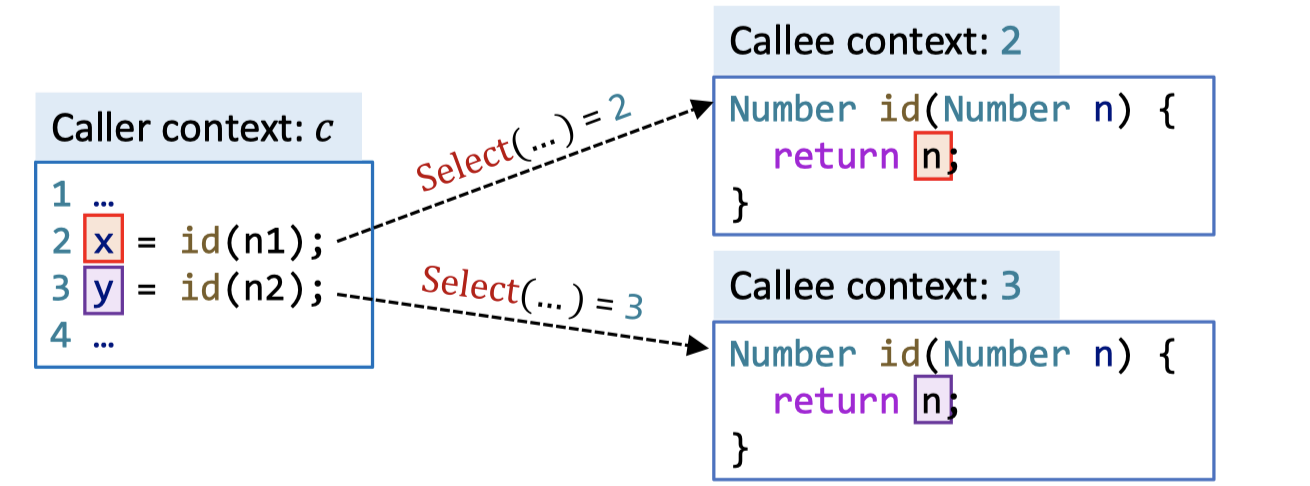## 上下文不敏感的指针分析存在的问题

void main() {
Number n1, n2, x, y;
n1 = new One();
n2 = new Two();
x = id(n1);
y = id(n2);
int i = x.get();
}
Number id(Number n) {
return n;
}
interface Number { int get(); }
class One implements Number { public int get() { return 1; }}
class Two implements Number { public int get() { return 2; }}


graph TD A("n1\n{o1}") --> C("n\n{o1, o2}") B("n2\n{o2}") --> C C --> D("x\n{o1, o2}") C --> E("y\n{o1, o2}")

graph TD A("n1\n{o1}") --> C("id(n1): n\n{o1}") B("n2\n{o2}") --> D("id(n2): n\n{o2}") C --> E("x\n{o1}") D --> F("y\n{o2}")

• 在动态执行过程中，一个方法可能在不同的调用上下文（calling contexts）中被调用多次。
• 在不同的调用上下文中，方法中的变量可能指向不同的对象。
• 在C.I.指针分析中，不同上下文中的对象通过返回值或方法副作用被混在一起传播到程序各处，导致了虚假数据流。

## 上下文敏感分析简介

### Call-Site Sensitivity

1 x = id(n1);
2 y = id(n2);
3 int i = x.get();
4
5 Number id(Number n) {
6     return n;
7 }


### Cloning-Based Context Sensitivity

graph TD A("n1\n{o1}") --> C(":n\n{o1}") B("n2\n{o2}") --> D(":n\n{o2}") C --> E("x\n{o1}") D --> F("y\n{o2}")

### Context-Sensitive Heap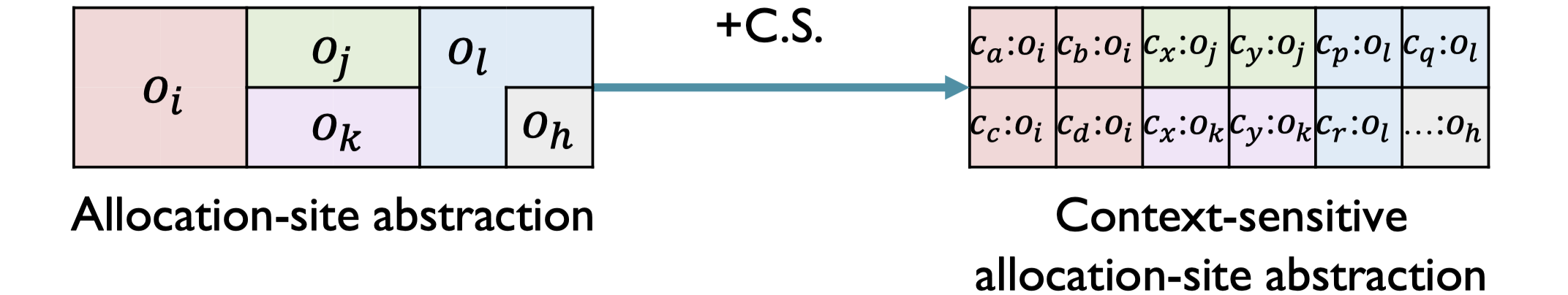• 在动态执行过程中，一个allocation site可能在不同的调用上下文中创建多个对象。
• 在同一个site创建的不同对象可能是由不同的数据流控制的——例如，将不同的值（值可能来自不同的方法调用）存储到对象的域中。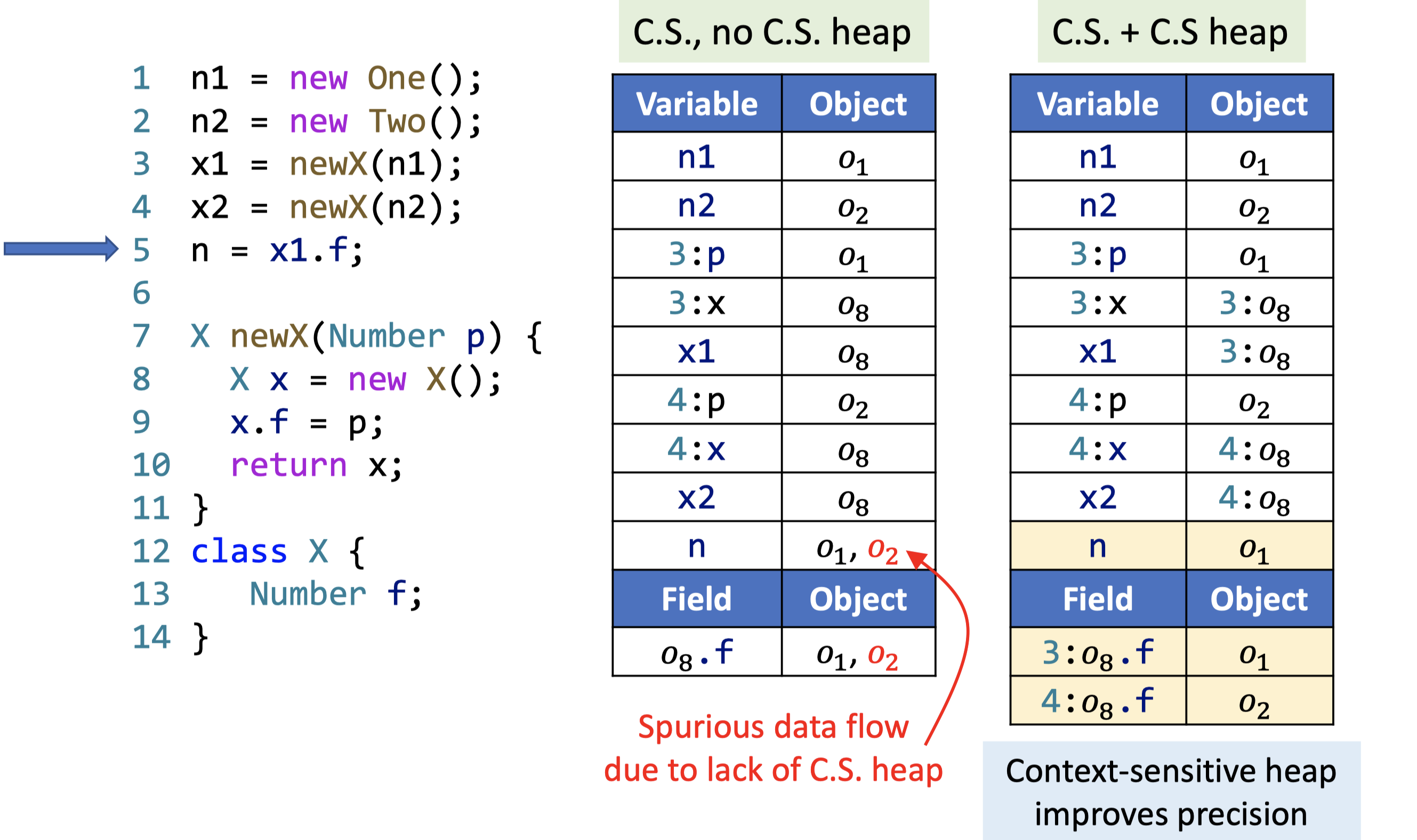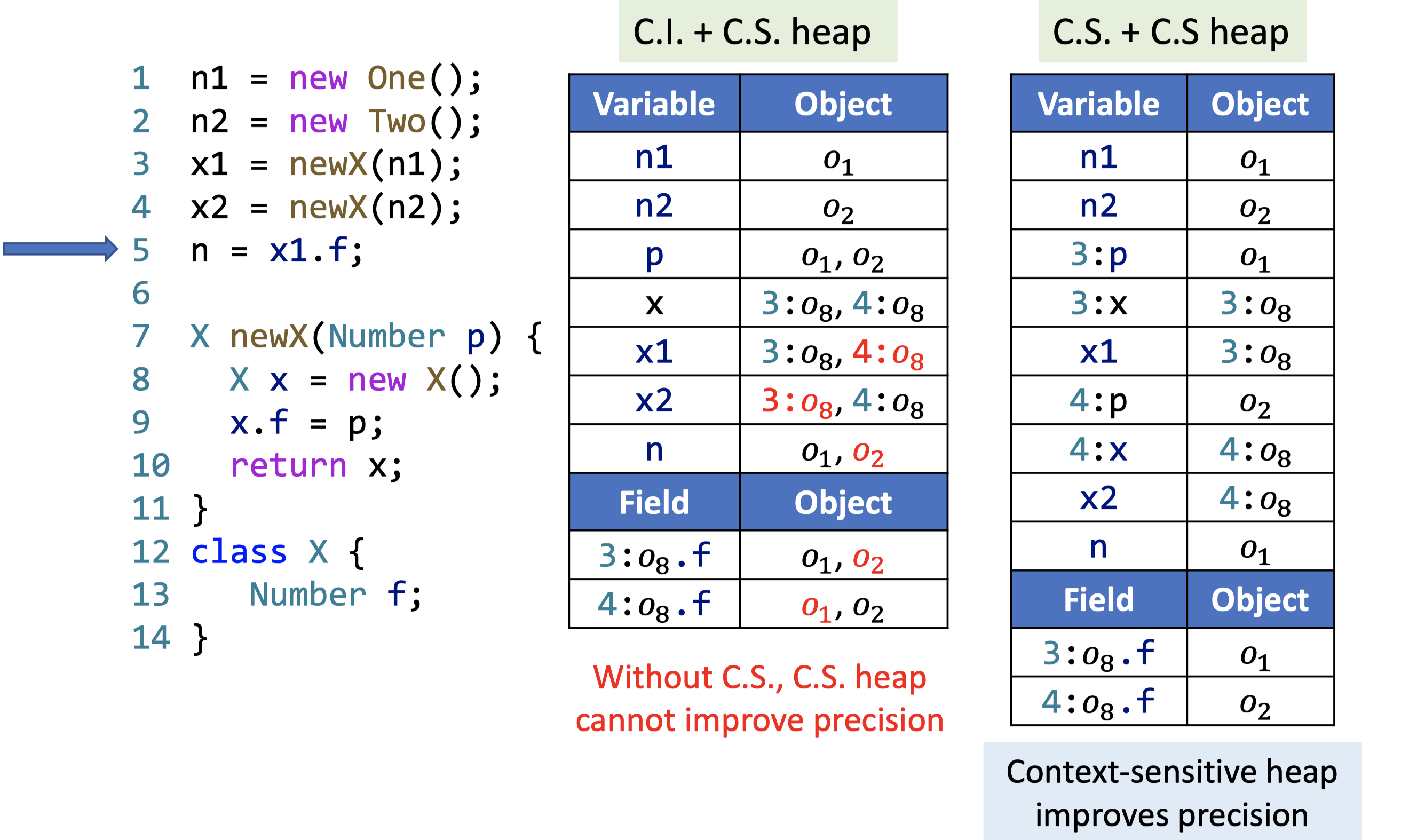## 上下文敏感的指针分析规则

• Context: $c, c’, c’’ \in C$
• Context-sensitive methods: $c:m \in C \times M$
• Context-sensitive variables: $c:x,\ c’:y \in C \times V$
• Context-sensitive objects: $c:o_{i},\ c’:o_{j} \in C \times O$
• Fields: $f, g \in F$
• Instance fields: $c: o_{i}.f,\ c’:o_{j}.g \in C \times O \times F$
• Context-sensitive pointers: $\text{CSPointer} = (C \times V) \cup (C \times O \times F)$
• Points-to relations: $pt: \text{CSPointer} \rightarrow P(C \times O)$

New i: x = new T() $\frac{}{c:o_{i} \in pt(c:x)}$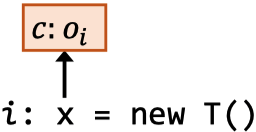Assign x = y $\frac{c’:o_{i} \in pt(c:y)}{c’:o_{i} \in pt(c:x)}$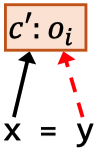Store x.f = y $\frac{c’:o_{i} \in pt(c:x),\ c’’:o_{j} \in pt(c:y)}{c’’:o_{j} \in pt(c’:o_{i}.f)}$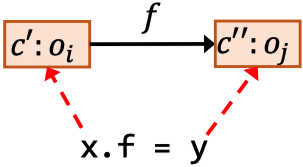Load y = x.f $\frac{c’:o_{i} \in pt(c:x),\ c’’:o_{j} \in pt(c’:o_{i}.f)}{c’’:o_{j} \in pt(c:y)}$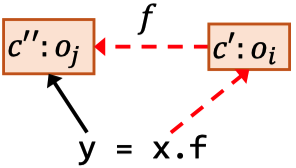Call l: r = x.k(a1, ..., an)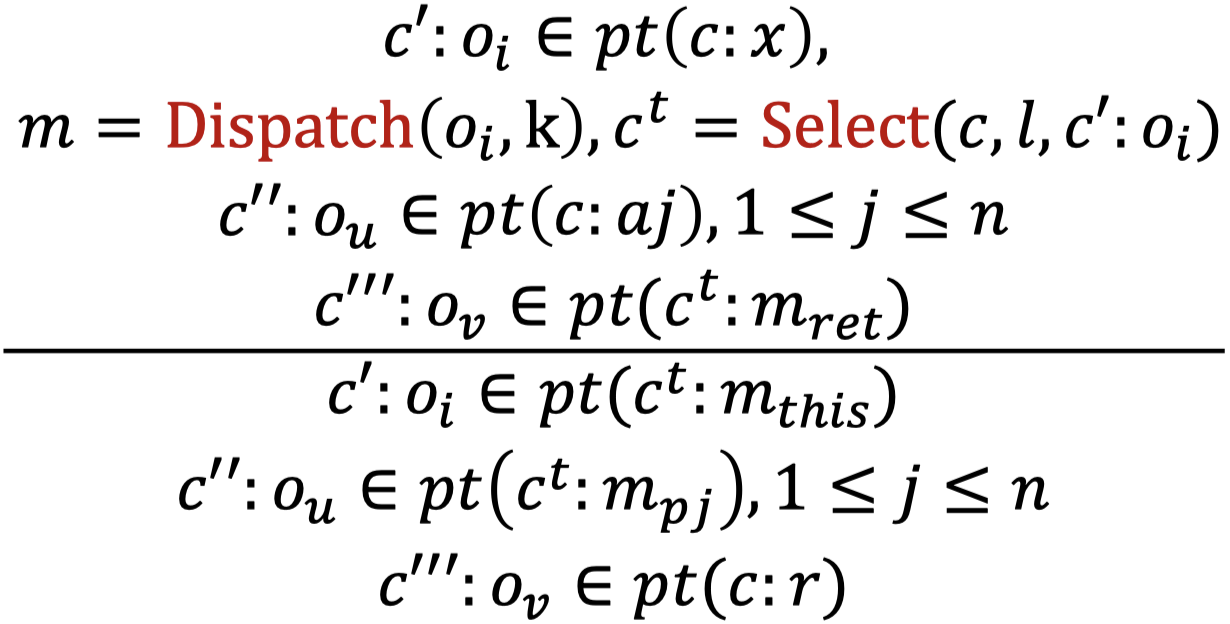-